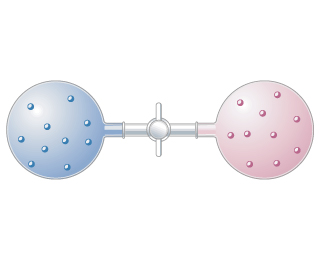# Problem: Two different gases occupy two separate bulbs. Consider the process that occurs when the stopcock separating the gases is opened, assuming the gases behave ideally.Is the process that occurs when the stopcock is opened a reversible one?

###### FREE Expert Solution

We are being asked to determine if the opening the stopcock is a reversible reaction.

Recall that a reversible process is a process in which the system and environment can be restored to the same initial states that they were in before the process occurred if we go back along the path of the process.

96% (152 ratings)###### Problem Details

Two different gases occupy two separate bulbs.Consider the process that occurs when the stopcock separating the gases is opened, assuming the gases behave ideally.

Is the process that occurs when the stopcock is opened a reversible one?

Frequently Asked Questions

What scientific concept do you need to know in order to solve this problem?

Our tutors have indicated that to solve this problem you will need to apply the Spontaneous Reaction concept. You can view video lessons to learn Spontaneous Reaction. Or if you need more Spontaneous Reaction practice, you can also practice Spontaneous Reaction practice problems.

What is the difficulty of this problem?

Our tutors rated the difficulty ofTwo different gases occupy two separate bulbs. Consider the ...as low difficulty.